Students can download 5th Maths Term 1 Chapter 2 Numbers Ex 2.5 Questions and Answers, Notes, Samacheer Kalvi 5th Maths Guide Pdf helps you to revise the complete Tamilnadu State Board New Syllabus, helps students complete homework assignments and to score high marks in board exams.

Tamilnadu Samacheer Kalvi 5th Maths Solutions Term 1 Chapter 2 Numbers Ex 2.5

Question 1.
Find the sum.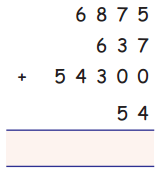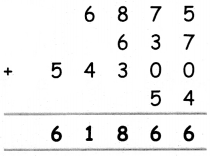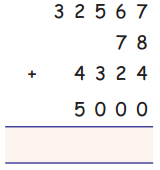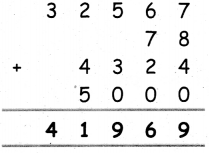Question 2.
a. 19732 + 24105 + 525 + 48
b. 241605 + 34788 + 5003 + 2052
c. 1000 + 250787 + 3574 + 43
d. 7 + 65 + 324 + 52342
a. 19732 + 24105 + 525 + 48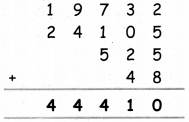b. 241605 + 34788 + 5003 + 2052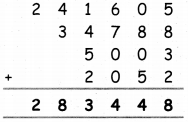c. 1000 + 250787 + 3574 + 43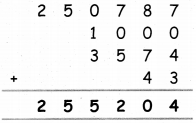d. 7 + 65 + 324 + 52342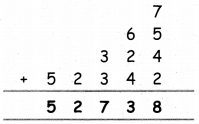Question 3.
Population of five villages in a town panchayat are 980; 3254; 4125;687;6786. What is the total population.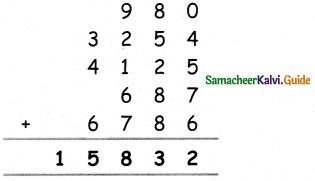Question 4.
Ramu bought some household things. The price list is given below. Find the total cost?
Fan – ₹ 3,250
Fridge – ₹ 26,437
Television – ₹ 18,520
Iron box – ₹ 940
Cot – ₹ 15,520
Fan – ₹ 3,250
Fridge – ₹ 26,437
Television – ₹ 18,520
Iron box – ₹ 940
Cot – ₹ 15,520
Total cost = ₹ 64667Question 5.
A day’s sales of different vegetables in a vegetable shop is as follows: Brinjals ₹4,500, Tomato ₹7,800, Onion ₹ 26,500, Potato ₹ 7,825 and Beetroot ₹825. Find the total amount of sales.